• dahoa
In summary, the moment is a load and bending stress that is exerted on a beam when it is subjected to an external load. The bending stress is the stress that is exerted on a beam when it is subjected to an external load.f

#### dahoa

Hi .. for a simple beam with uniformly distributed load and moment formula of w * ln /8 at center.. is it independent of thickness of the beam such that even if a beam is 1 meter depth by half meter width compare to beam half or even twice its size..the formula and moment is still the one for a given length and load?

Hi .. for a simple beam with uniformly distributed load and moment formula of w * ln /8 at center.. is it independent of thickness of the beam such that even if a beam is 1 meter depth by half meter width compare to beam half or even twice its size..the formula and moment is still the one for a given length and load?
Of course.
The characteristics of the beam only affect its ability to withstand a given load. The load is whatever it is regardless.
Does your weight depend on the details of the floor you stand on?

Of course.
The characteristics of the beam only affect its ability to withstand a given load. The load is whatever it is regardless.
Does your weight depend on the details of the floor you stand on?

But weight is weight. unless you mean it is ok to ask a person what is his/her moments on a 3 meters fixed beam?

I mean even if you are standing in a 1 mile thick iron.. the moment is the same as a small wooden beam? What principle produce the same moment/bending?

But weight is weight. unless you mean it is ok to ask a person what is his/her moments on a 3 meters fixed beam?
Once you know where on the beam they stand, yes.

Once you know where on the beam they stand, yes.

I added this that you missed...

I mean even if you are standing in a 1 mile thick iron.. the moment is the same as a small wooden beam? What principle produce the same moment/bending?

the same moment/bending?
Same moment, different bending.

Hi .. for a simple beam with uniformly distributed load and moment formula of w * ln /8 at center.. is it independent of thickness of the beam such that even if a beam is 1 meter depth by half meter width compare to beam half or even twice its size..the formula and moment is still the one for a given length and load?
I think, the bending moment is independent of the cross sectional dimensions of the beam.
Bending moment is just a resisting moment offered by the beam.Bending moment will be equal to the moment due to the applied loads as long as the bending stress induced is less than the yield stress(ductile) or fracture strength(brittle).Once it reaches the yield stress, the beam fails(It can't resist anymore).
Assuming same material,same length of the beams, same load applied,
for a beam with higher section modulus(roughly represents cross sectional area) lesser bending stress is induced but for a beam with lower section modulus(smaller cross section), higher bending stress is induced.
So the stress in the beam with lower section modulus can easily reach the failure stress with a lesser load.so they fail easily than the thicker beams.

Last edited:
offered by the beam.
Offered to the beam, surely?

Same moment, different bending.

Whats the best definitions of the difference between moment and bending anyone has come across?

Whats the best definitions of the difference between moment and bending anyone has come across?
It's not that subtle a difference. The moment describes the load applied and its distribution; the bending is what results, and that will depend on attributes of the beam.
Generically, these are called stress and strain. If you hang a weight from a rope, the weight is the stress and the stretch of the rope is the strain.

Offered to the beam, surely?
I think it is offered by the beam.Because bending stress is a resistance or opposition by the beam to the externally applied loads and moments at a cross section.
On one half of the cross section, the stress is tensile (surfaces are elongated) so this produces a net resisting tensile load on that half.On the other it is compressive so net compressive resisting load.
These two equal opposite parallel loads cause a resisting couple .This couple is called bending moment.(FYI:I can't understand the difference between couple and moment)
Afterall in static equilibrium condition, for every applied load or moment there should be equal an opposite reactions else the beam won't be stationary.
Initialy when you apply load on an unloaded beam, it starts bending.During bending, the internal resisting moment starts increasing and when it becomes equal to the moment due to external applied load, the bending stops and the beam is held at that position(beam comes to rest because net moment acting is zero).This is the state of static equilibrium which we usualy study about.

Whats the best definitions of the difference between moment and bending anyone has come across?
Initialy a beam will be flat(straight).When you apply transverse shear loads(loads perpendicular to the longitudinal axis of the beam) on the beam, the loads cause moments (rotating effects of the loads).These moments try to rotate the beam but since the beam resists the rotation(by applying a resisting moment opposite to this external moment), its shape will change.This process in which the shape of the beam changes is called bending and the rotating effect of the applied loads is called moment(external moments due to applied loads).The resisting moment by the beam is called bending moment.

bending stress is a resistance or opposition by the beam to the externally applied loads
Ok. But in a static arrangement it is equal and opposite to the applied load's moment, so is dependent only on that load, not on any characteristics of the beam.

Ok. But in a static arrangement it is equal and opposite to the applied load's moment, so is dependent only on that load, not on any characteristics of the beam.
Bending stress is dependent on the cross sectional area or section modulus(higher cross sectional area, lower stress).But the net reaction load caused by the stress is independent of the cross sectional area because the ne resisting force is F=(Stress)×(Area).This means lower stress over a larger cross section and higher stress over a smaller cross section will give the same resisting force and hence the same resisting couple(bending moment) irrespective of the cross section.
(Its because of this reason,in case of axial loading we assume the magnitude of the external load as the magnitude of the resisting force and calculate the magnitude of the stress by using STRESS=EXTERNAL LOAD÷AREA OF CROSS SECTION
but the actual formula is stress=Resisting force÷area)
The rsisting moment depend only on the moments caused by the external loads.
But the stress induced will depend on the dimensions of the beam also.

Last edited:
Bending stress is dependent on the cross sectional area
The original question is regarding bending moment, not stress. However the stress is distributed in the beam, it results in a resistive moment equal and opposite to the applied moment, and this is independent of the beam characteristics.

The original question is regarding bending moment, not stress. However the stress is distributed in the beam, it results in a resistive moment equal and opposite to the applied moment, and this is independent of the beam characteristics.
Yes.I think it is right.
Earlier, I said that" bending moment is the resisting moment"(i.e.it is applied by the bar).But it might be wrong.I thought of two possibilities.
I think the reason behind calling the resisting moment as the bending moment is that the magnitude of the resisting moment at any cross section will be equal to the resultant moment due to all the externally applied loads and couples about that cross section(but opposite in direction since it resists them).
i.e., Since its magnitude is equal to the net external moment that tries to bend the beam about that cross section, it is called the bendding moment.
(OR THE OTHER POSSIBILITY MIGHT BE)
Bending moment and resisting moment might be two different things.As in the case of normal tensile stress(stress=resisting force÷Area), the formula for bending stress might be "resisting moment÷section modulus" but since the magnitude of the resisting moment is equal to the net external bending moment we might be using the formula "bending moment÷section modulus"
These might even be wrong.
what do you think is right?

Last edited: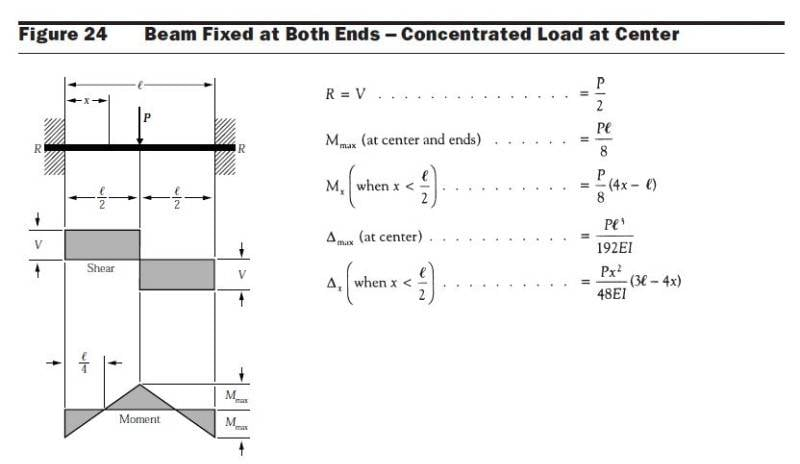How is moment of P*length/8 exactly derived above? why divided by 8 and not 6 or others?

Does it depend on the gravitational constant or any Spacetime feature?

What kind of spacetime where there is no moment even if there is a loading?

#### Attachments

How is moment of P*length/8 exactly derived above?
To compute the moment at some point, we only consider those loads between one end (the left, say) and the point.
Since P is uniformly spread along l, only P/2 is applied to the left of the midpoint.
We could find its moment about the midpoint by an integral, but it is easier than that. We can just look at the average point of application. This is halfway between the left end and the midpoint of the beam, so at l/4 from the midpoint.
The moment is therefore (P/2)(l/4)=Pl/8.

To compute the moment at some point, we only consider those loads between one end (the left, say) and the point.
Since P is uniformly spread along l, only P/2 is applied to the left of the midpoint.
We could find its moment about the midpoint by an integral, but it is easier than that. We can just look at the average point of application. This is halfway between the left end and the midpoint of the beam, so at l/4 from the midpoint.
The moment is therefore (P/2)(l/4)=Pl/8.
But I think it is not a distributed load, it is point load.

View attachment 228308

How is moment of P*length/8 exactly derived above? why divided by 8 and not 6 or others?

Does it depend on the gravitational constant or any Spacetime feature?

What kind of spacetime where there is no moment even if there is a loading?
Beams with fixed supporsts at both the ends are called statically indeterminate beams.Because, you can't directly find the reaction forces and reaction moments at the supports.In this case you may easily find the reaction forces(R=P/2) because the load acts exactly at the midpoint.
Iam not sure about how to find the reaction moments.Refer some books.

To compute the moment at some point, we only consider those loads between one end (the left, say) and the point.
Since P is uniformly spread along l, only P/2 is applied to the left of the midpoint.
We could find its moment about the midpoint by an integral, but it is easier than that. We can just look at the average point of application. This is halfway between the left end and the midpoint of the beam, so at l/4 from the midpoint.
The moment is therefore (P/2)(l/4)=Pl/8.

But to get moment.. why do you need to multiply the load by the length.. it's as if moment is load in circle or with circular radius?

But I think it is not a distributed load, it is point load.
Sorry- didn't read the diagram title.

As you say, it is not, strictly speaking, determinable, so they are assuming symmetry.
If the reaction force at the left is V and the moment is M then the moment at offset x is Mx=M-Vx=-ky" for some constant k.
(I take anticlockwise as positive.)
Integrating: ky'=Mx-½Vx2+c.
By fixture this is 0 at x=0 and by symmetry 0 at x=l/2:
c=0, M=Vl/4.
So Mx=V(l/4-x)=P/8(l-4x)
Looks like the book takes clockwise as positive.

•dahoa and Mohankpvk
But to get moment.. why do you need to multiply the load by the length.. it's as if moment is load in circle or with circular radius?
My initial post was wrong - please read post #22. But to answer your question above, that's what a moment is: it is the applied force multiplied by its perpendicular displacement from the axis.

I detect a troll-like inclination to involve pseudo-philosophical irrelevancies.
"Epistemological" means pertaining to a theory of knowledge. "Ontological", pertaining to the essential nature of things. Those terms could be applied to a line of reasoning or philosophical stance but not to a concept such as moment or force.

I have explained how the expression for Mx is obtained.
I will not waste my time further on this thread until you ask serious scientific questions.

I am studying structural engineering.. I'm just figuring out how certain things are derived so I can understand them at first principles. Thanks a lot for your help. I'm digesting it. So I guess moment is like acceleration due to gravity. If we are standing on ground, we are not falling although there is a tendency.. so likewise with moment.. if the beam is made of diamond.. there is tendency too but stopped from full expression.

I guess moment is like acceleration due to gravity. If we are standing on ground, we are not falling although there is a tendency
Indeed, and if you take away whatever is preventing motion then a force, such as gravity, produces linear acceleration, whereas a moment produces angular acceleration.

•Mohankpvk and dahoa
I've been solving something for a month as well as reading half a dozen structural engineering books. Maybe you can help.

Pinned and fixed supports can affect the moments and reactions.. for example.. a simple support pinned and fixed has the following moments...

pinned supports: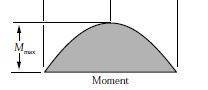fixed supports: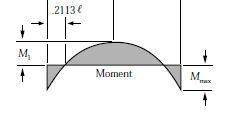You will noticed fixed support have more moments at the supports and less moments at midspan..

In structuring engineering.. you get the loads of the beam by the tributary areas of the floors or slabs.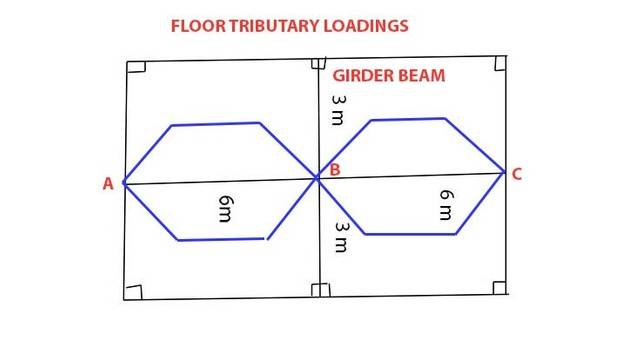In 3 pinned supports.. the formulas for the reactions are: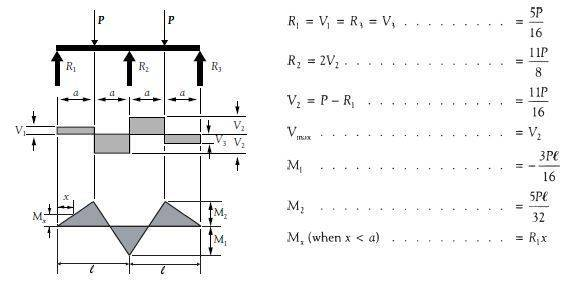However, I can't get reference what would be the reactions if all the supports were fixed instead of pinned. The ends (A & C) would be have reactions and contributions to the load carrying if fixed than if they were all pinned. Any idea how to derive formulas for the fixed support cases?

Thank you.

#### Attachments

what would be the reactions if all the supports were fixed instead of pinned.
If an intermediate support is fixed then what happens on side of it cannot affect what happens on the other side, so the problem splits into two separate problems.

If an intermediate support is fixed then what happens on side of it cannot affect what happens on the other side, so the problem splits into two separate problems.

If R2 which is in the middle is fixed.. and R1 and R3 are pinned.. there are more reactions at R2. Whereas if R1 and R3 are fixed.. R1 and R3 can take more reactions. You are saying R1 and R2, R2 and R3 are separate problems? Hmm... Then let's just take R1 and R2.. what happened if R1 is fixed instead of Pinned?

But then the case is about 2 point loads at either sides and R1 formula is 5P/16 or 0.3125P, whereas R2 formula is 11P/8 or 1.375P. Now if all are fixed.. R1 and R3 should take more loads maybe 0.65P or sorta. I want formula to compute it.

Is it clear to you that, if the ends are pinned, the reaction forces are statically determinate? Is it also clear to you that, if the ends are "built in," the reaction forces and moments are statically indeterminate? Do you know the difference?

Is it clear to you that, if the ends are pinned, the reaction forces are statically determinate? Is it also clear to you that, if the ends are "built in," the reaction forces and moments are statically indeterminate? Do you know the difference?

If the ends are fully moment connected (100% fixed).. why can't you determine the reaction forces and moments?

You are saying R1 and R2, R2 and R3 are separate problems?
Yes.

If the ends are fully moment connected (100% fixed).. why can't you determine the reaction forces and moments?
I think its because, there will be more unknowns than the number of static equilibrium equations that can be applied.If both ends of a beam are fixed, you have to find two reaction meoments(one at each end) and two reaction vertical forces(one at each end).But you have only two static equilibrium equations.(summation of vertical forces=0 and summation of moment about any point=0).

I think its because, there will be more unknowns than the number of static equilibrium equations that can be applied.If both ends of a beam are fixed, you have to find two reaction meoments(one at each end) and two reaction vertical forces(one at each end).But you have only two static equilibrium equations.(summation of vertical forces=0 and summation of moment about any point=0).
No, there's more to it than that.
Consider the simplest case: no load applied, weightless beam, ends at same height and fixed horizontally.
If this is statically indeterminate then there must be a nontrivial solution, i.e., one in which the end supports apply equal and opposite nonzero vertical forces. Correspondingly there will be nonzero torques so that equilibrium is maintained.
For a theoretical rigid beam that would all be possible, but this field of study considers beams to be flexible. The beam undergoes a deflection y=y(x).

Suppose the supports give a force F up at the left and down at the right, and anticlockwise torques T, T' respectively.
Taking moments at offset x from the left:
ky"=-T+Fx
Integrating, and using y(0)=0:
ky'=-Tx+Fx2/2
Integrating, and using y'(0)=0:
ky=-Tx2/2+Fx3/6
Now we use the same constraints at the right hand end (x=L):
0=-TL+FL2/2
0=-TL2/2+FL3/6
When the smoke clears:
T=F=0.

So it is not indeterminate after all.
(I was wrong to agree to that earlier without checking.)

•dahoa and Mohankpvk
anyway.. I have been trying to derive these for a month but couldn't... in the following:

View attachment 228381

How is R1=R3=5P/16 and R2= 11P/8 exactly derived?
Try applying the procedure I posted at #22 and #34.